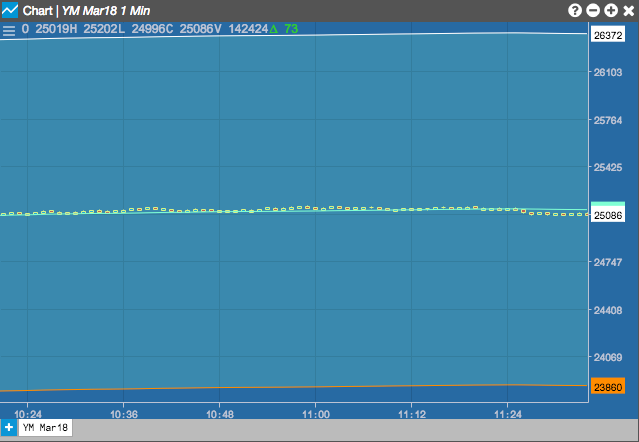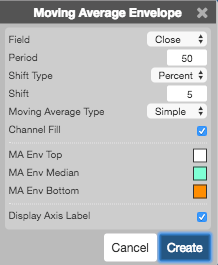Charts

# Moving Average Envelope (MAE)

The Moving Average Envelope creates an envelope around a moving average to find overbought or oversold conditions, to smooth the price trend and as an indicator of price breakouts. The moving average envelope uses a moving average as the center line with a lower and upper bands added a percetnage above and below the center line.## Configuration Options• Field: Price or combination of prices to use as the base for average calculations. Possible values include:
• Open
• High
• Low
• Close
• HL/2 $$\left ( \frac{High + Low}{2} \right )$$
• HLC/3 $$\left ( \frac{High + Low + Close}{3} \right )$$
• HLCC/4 $$\left ( \frac{High + Low + Close + Close}{4} \right )$$
• OHLC/4 $$\left ( \frac{Open + High + Low + Close}{4} \right )$$
• Period: Number of bars to use in the calculations.
• Shift Type: Type of offset (percent or points)
• Shift: Percentage or number of points to offset above or below the average.
• Moving Average Type: Type of moving average to use in the calculations:
• Simple
• Exponential
• Time Series
• Triangular
• Variable
• VIDYA
• Weighted
• Welles Winder
• Hull
• Double Exponential
• Triple Exponential
• Channel Fill: Whether to shade the area between the top and bottom lines.
• Color Selectors: Colors to use for graph elements.
• Display Axis Label: Whether to display the most recent value on the Y axis.

## Formula

Envelopes start with any moving average type (see Moving Averages for formulas) and then creates an offset x% above or below the average or an offset Y points above or below the average.

• For percentage envelopes:

Upper band = moving average + x%(moving average)
Lower band = moving average – x%(moving average)
• For points envelopes:

Upper band = moving average + constant
Lower band = moving average – constant